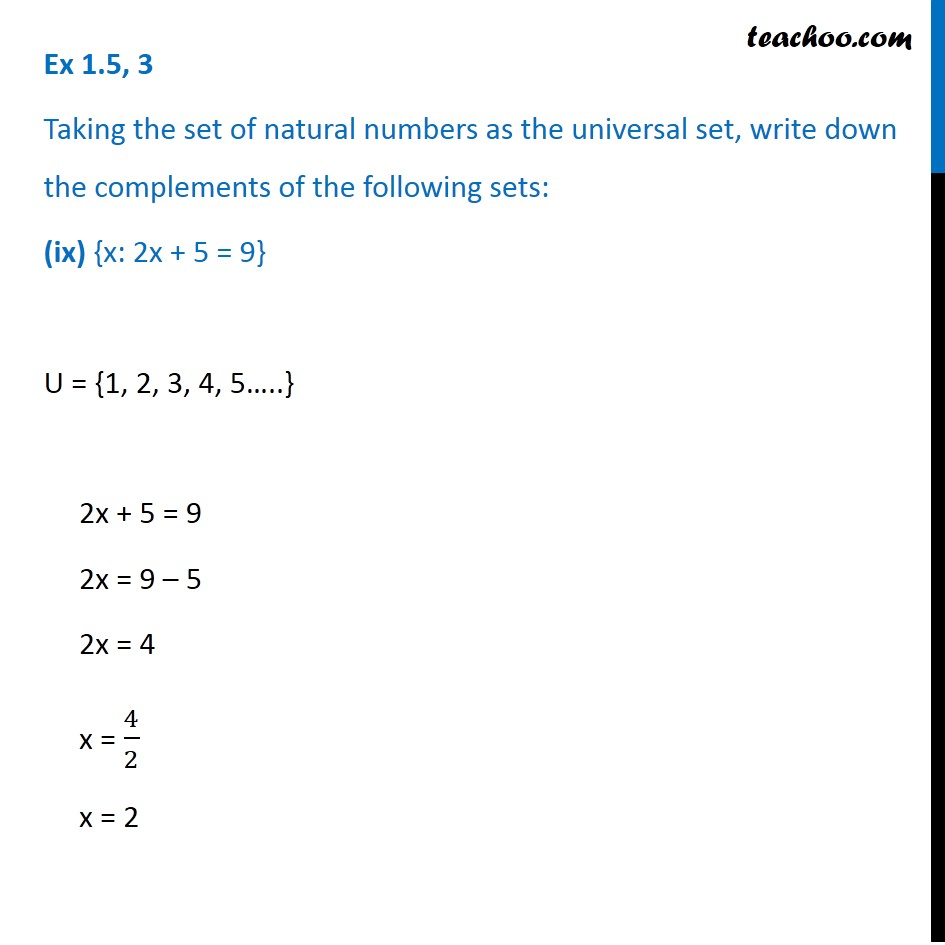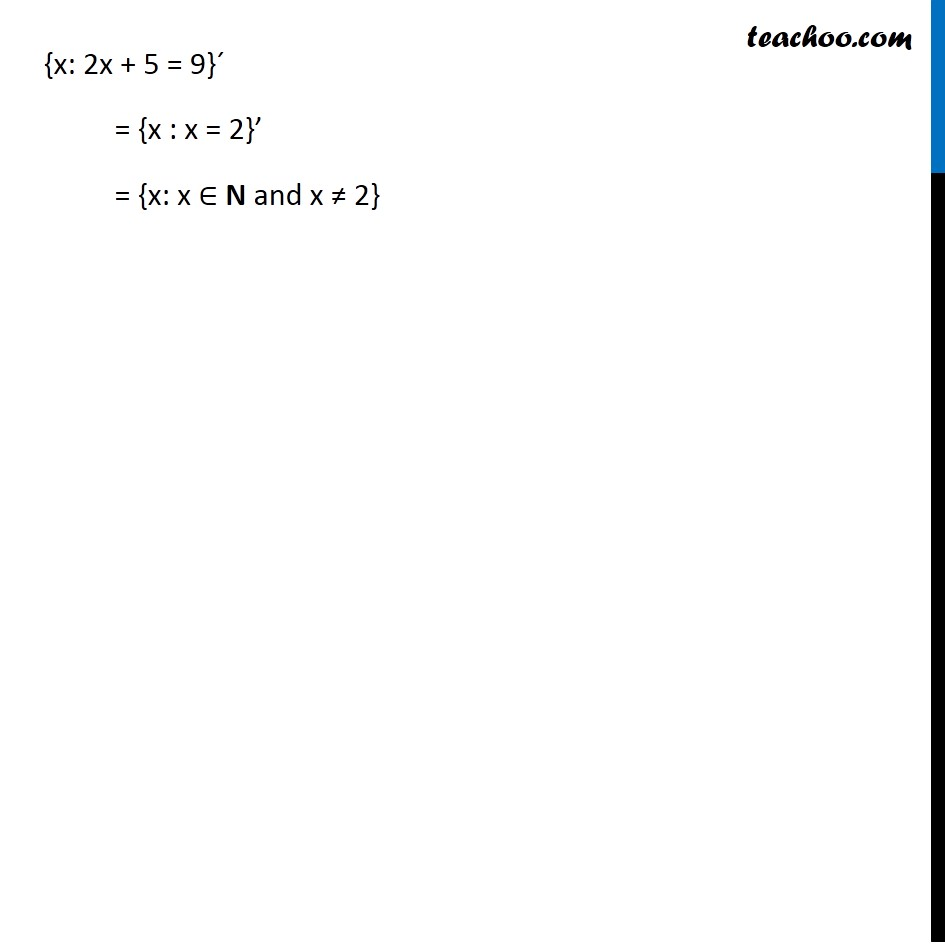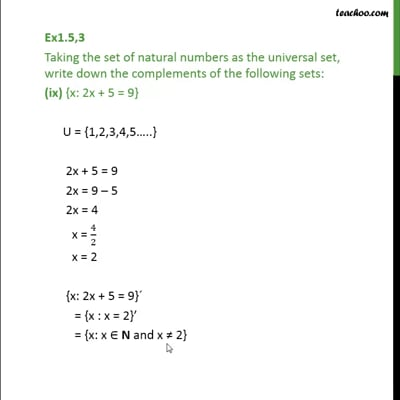Ex 1.5

Chapter 1 Class 11 Sets (Term 1)
Serial order wiseThis video is only available for Teachoo black users

### Transcript

Ex 1.5, 3 Taking the set of natural numbers as the universal set, write down the complements of the following sets: (ix) {x: 2x + 5 = 9} U = {1, 2, 3, 4, 5…..} 2x + 5 = 9 2x = 9 – 5 2x = 4 x = 4/2 x = 2 {x: 2x + 5 = 9}´ = {x : x = 2}’ = {x: x ∈ N and x ≠ 2}